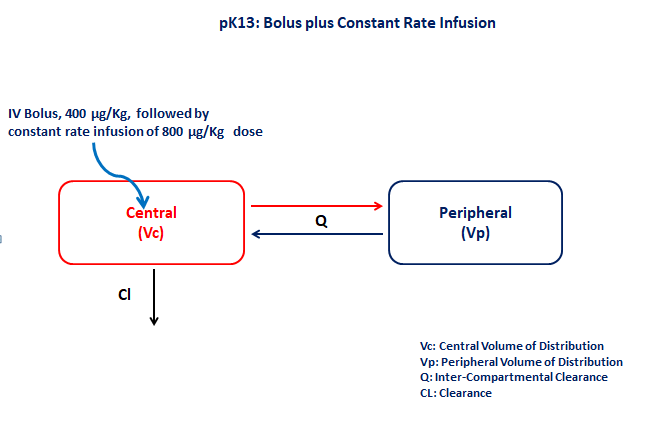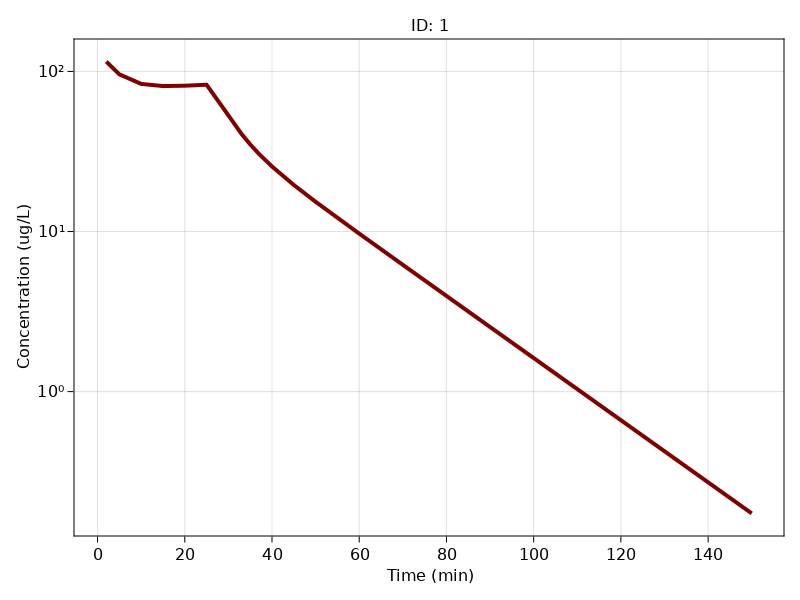# PK13 - Bolus plus constant rate infusion

### Background

• Structural model - Two compartment model with first order elimination

• Route of administration - IV-Bolus and IV-Infusion given simultaneously

• Dosage Regimen - 400 μg/kg IV-Bolus and 800 μg/kg IV-Infusion for 26 mins at time=0

• Number of Subjects - 1### Learning Outcome

• Write the differential equation for a two-compartment model in terms of Clearance and Volume

• Simulate data for both a bolus dose followed by a constant rate infusion regimen

### Objective

The objective of this exercise is to simulate data from a bolus followed by a constant rate infusion using differential equation model.

### Libraries

Call the "necessary" libraries to get started

using Random
using Pumas
using PumasUtilities
using CairoMakie


### Model

The given data follows a two compartment model in which the IV Bolus and IV-Infusion are administered at time=0

pk_13           = @model begin
desc        = "Two Compartment Model"
timeu       = u"minute"
end

@param begin
"Clearance (L/min/kg)"
tvcl        ∈ RealDomain(lower=0)
"Volume of Central Compartment (L/kg)"
tvvc        ∈ RealDomain(lower=0)
"Inter-compartmental Clearance (L/min/kg)"
tvq         ∈ RealDomain(lower=0)
"Volume of Peripheral Compartment (L/kg)"
tvvp        ∈ RealDomain(lower=0)
Ω           ∈ PDiagDomain(4)
"Proportional RUV"
σ²_prop     ∈ RealDomain(lower=0)
end

@random begin
η           ~ MvNormal(Ω)
end

@pre begin
Cl          = tvcl * exp(η)
Vc          = tvvc * exp(η)
Q           = tvq * exp(η)
Vp          = tvvp * exp(η)
end

@dynamics begin
Central'    = -(Cl/Vc)*Central -(Q/Vc)*Central +(Q/Vp)*Peripheral
Peripheral' = (Q/Vc)*Central -(Q/Vp)*Peripheral
end

@derived begin
cp          = @. Central/Vc
"""
Observed Concentrations (ug/L)
"""
dv          ~ @. Normal(cp, sqrt((cp*σ²_prop)^2 + σ²_add^2))
end
end

PumasModel
Parameters: tvcl, tvvc, tvq, tvvp, Ω, σ²_prop, σ²_add
Random effects: η
Covariates:
Dynamical variables: Central, Peripheral
Derived: cp, dv
Observed: cp, dv


### Parameters

• $Cl$ - Clearance of central compartment (L/min/kg)

• $Vc$ - Volume of central compartment (L/kg)

• $Q$ - Inter-compartmental clearance (L/min/kg)

• $Vp$ - Volume of peripheral compartment (L/kg)

• $Ω$ - Between Subject Variability

• $σ$ - Residual Unexplained Variability

param = ( tvcl        = 0.344708,
tvvc        = 2.8946,
tvq         = 0.178392,
tvvp        = 2.18368,
Ω           = Diagonal([0.0, 0.0, 0.0, 0.0]),
σ²_prop     = 0.0571079,

(tvcl = 0.344708, tvvc = 2.8946, tvq = 0.178392, tvvp = 2.18368, Ω = [0.0 0
.0 0.0 0.0; 0.0 0.0 0.0 0.0; 0.0 0.0 0.0 0.0; 0.0 0.0 0.0 0.0], σ²_prop = 0


### Dosage Regimen

• Single dose of 400 μg/kg given as IV-Bolus at time=0

• Single dose of 800 μg/kg given as an IV-Infusion for 26 mins at time=0

ev1  = DosageRegimen(400, time = 0, cmt = 1)
ev2  = DosageRegimen(800, time = 0, cmt = 1, rate = 30.769)
ev3  = DosageRegimen(ev1, ev2)
sub1 = Subject(id = 1, events = ev3)

Subject
ID: 1
Events: 3


### Simulation

We will simulate the plasma concentration at the pre specified time points.

Random.seed!(123)
sim_sub1 = simobs(pk_13, sub1, param,
obstimes=[2,5,10,15,20,25,30,33,35,37,40,45,50,60,70,90,110,120,150])


### Visualization

sim_plot(pk_13, [sim_sub1],
observations = :cp,
linewidth = 4,
color = :maroon,
axis = (xlabel = "Time (min)",
ylabel="Concentration (ug/L)",
yscale = log10, xticks = 0:20:160))